# Understanding Maxwell Equations

Published  July 11, 2019   0
S
Author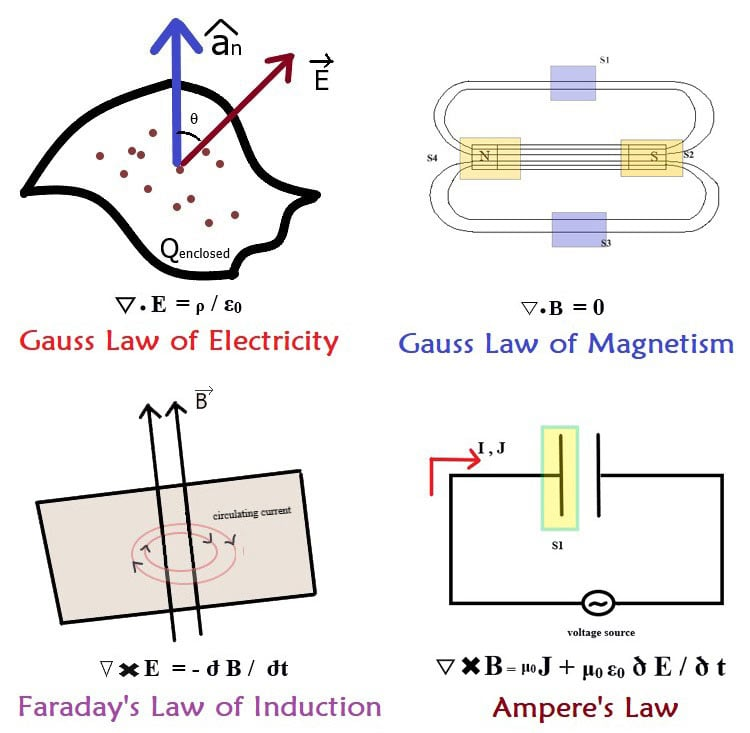Maxwell equations are the fundamentals of Electromagnetic theory, which constitutes a set of four equations relating the electric and magnetic fields. Instead of listing out the mathematical representation of Maxwell equations, we will focus on what is the actual significance of those equations in this article. The Maxwell’s First and Second equation deals with static electric fields and static magnetic fields respectively. The Maxwell’s Third and Fourth equation deals with changing magnetic fields and changing electric fields respectively.

The Maxwell equations are:

1. Gauss Law Of Electricity
2. Gauss Law of Magnetism
4. Ampere’s Law

### 1. Gauss Law Of Electricity

This law states that the Electric Flux out of a closed surface is proportional to the total charge enclosed by that surface. The Gauss law deals with the static electric field.Let us consider a positive point charge Q. We know that the electric flux lines are directed outwards from the positive charge.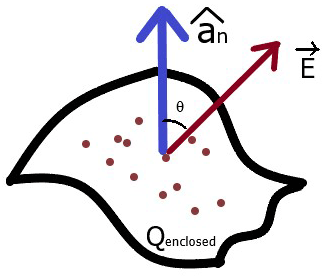Let us consider a closed surface with Charge Qenclosed in it. The Area Vector is always chosen Normal to it because it represents the orientation of the surface. Let the angle made by the Electric field vector with the area vector be θ.

The Electric Flux ψ is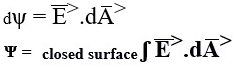The reason for choosing the dot product is that we need to calculate how much electric flux passes through the surface represented by a normal area vector.

From coulombs law, we know that the Electric Field (E) due to a point charge is Q/ 4πε0r2.

Considering a spherical symmetry, the Integral form of Gauss law is: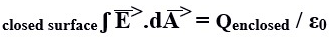Therefore the Electric Flux  Ψ = Qenclosed / ε0

Here the Qenclosed represents the vector sum of all the charges inside the surface. The region enclosing the charge can be of any shape but to apply Gauss law, we have to select a Gaussian surface which is symmetric and has uniform charge distribution. The Gaussian surface can be cylindrical or spherical or a plane.

To derive its Differential form, we need to apply Divergence theorem.

The above equation is the differential form of Gauss Law or Maxwell equation I.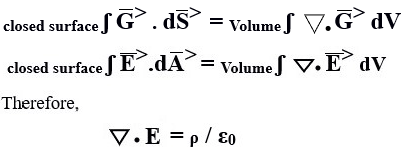In the above equation, ρ represents the Volume charge density. When we have to apply the Gauss law to a surface with a line charge or surface charge distribution, it is more convenient to represent the equation with charge density.

Therefore we can infer that the Divergence of an Electric field over a closed surface gives the amount of charge (ρ) enclosed by it. By applying divergence to a vector field, we can know whether the surface enclosed by the vector field is acting as a source or sink.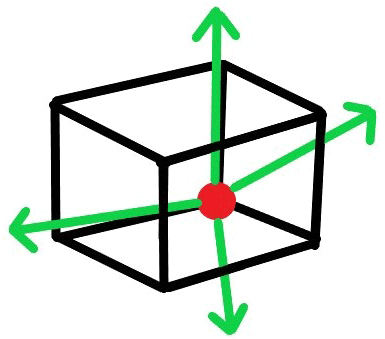Let us consider a cuboid with a positive charge as shown above. When we apply divergence to the electric field coming out the box (cuboid), the result of the mathematical expression tells us that the box (cuboid) considered acts as a source for the electric field computed. If the result is negative, it tells us that the box acts as a sink i.e. the box encloses a negative charge in it. If the divergence is Zero, it means that there is no charge in it.

From this, we could infer that electric monopoles exist.

### 2. Gauss Law of Magnetism

We know that the magnetic flux line flows from North pole to south pole externally.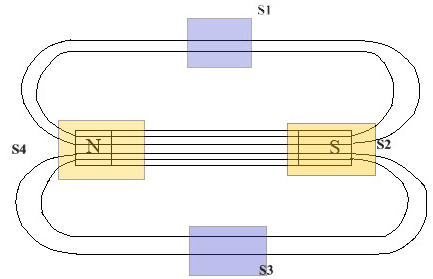Since there are magnetic flux lines due to a permanent magnet, there will be an associated magnetic flux density (B) of it. When we apply divergence theorem to surface S1, S2, S3 or S4, we see that the number of flux lines coming in and going out of the surface selected remains the same. Therefore the result of the divergence theorem is Zero. Even in the surface S2 and S4, the divergence is zero, which means that neither the north pole nor south pole individually acts a source or sink like the electric charges. Even when we apply divergence of the magnetic field (B) due to a current carrying wire, it turns out to be zero.

The integral form of Gauss law of Magnetism is: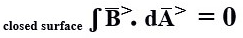The differential form of Gauss law of Magnetism is: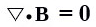From this, we could infer that magnetic monopoles do not exist.

### 3. Faraday’s Law of Induction

Faraday’s law states that when there is a change in magnetic flux (changing with respect to time) linking a coil or any conductor, there will be an EMF induced in the coil. Lenz’s stated that the EMF induced will be in a direction such that it opposes the change in magnetic flux producing it.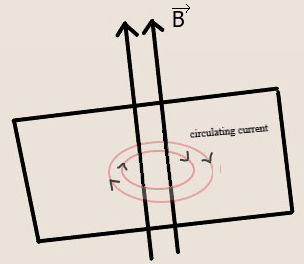In the above illustration, when a conducting plate or a conductor is brought under the influence of a changing magnetic field, circulating current is induced in it. The current is induced in such a direction that the magnetic field produced by it opposes the changing magnetic that created it. From this illustration, it is clear that changing or varying magnetic field creates a circulating electric field.

`emf = - dϕ / dt`

We know that,

```ϕ = closed surface ʃ B . dS
emf = - (d/dt)  ʃ B . dS```

Electric Field E= V/d

`V=  ʃ E .dl`

Since the electric field is changing with respect to the surface (curl), there exists a potential difference V.

Therefore the integral form of Maxwell’s fourth equation is,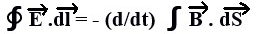By applying Stoke’s theorem,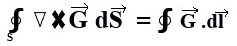The reason for applying Stoke’s theorem is that when we take a curl of a rotating field over a closed surface, the internal curl components of the vector cancels each other and this results in evaluating the vector field along the closed path.

Hence we can write that,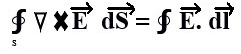The differential form of Maxwell’s equation is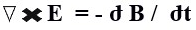From the above expression, it is clear that a magnetic field changing with respect to time produces a circulating Electric field.

Note: In electrostatics, the curl of an Electric field is zero because it emerges radially outwards from the charge and there is no rotating component associated with it.

### 4. Ampere’s Law

Ampere’s law states that when an electric current flows through a wire, it produces a magnetic field around it. Mathematically, the line integral of the magnetic field around a closed loop gives the total current enclosed by it.

` ʃ B .dl = μ0 Ienclosed`

Since the magnetic field curls around the wire, we can apply Stoke’s theorem to Ampere’s law.

Therefore the equation becomes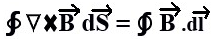We can represent the current enclosed in terms of current density J.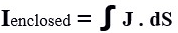B =  μ0 H by using this relation, we can write the expression as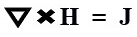When we apply divergence to the curl of a rotating vector field, the result is zero. It is because the closed surface doesn’t act as a source or sink i.e. the number of flux coming in and going out of the surface is the same. This can be mathematically represented as,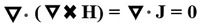Let us consider a circuit as illustrated below.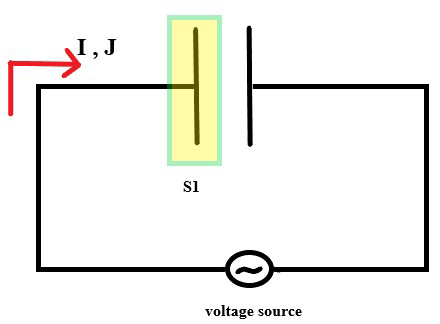The circuit has a capacitor connected to it. When we apply divergence in the region S1, the result shows that it is non-zero. In mathematical notation,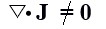There is a current flow in the circuit but in the capacitor, the charges get transferred due to changing electric field across the plates. So physically the current does not flow through it. Maxwell coined this changing electric flux as Displacement Current (JD). But Maxwell coined the term Displacement Current (JD) considering the symmetry of the Faraday’s law i.e if a magnetic field changing in time produces an Electric field then by symmetry, changing electric field produces a magnetic field.

The curl of magnetic field intensity (H) in the region S1 is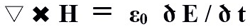The integral form of Maxwell’s fourth equation can be expressed as: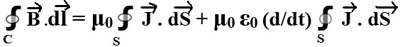The differential form of Maxwell’s fourth equation is: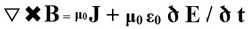All these four equations either in the integral form or differential form put together are called as Maxwell’s Equation.